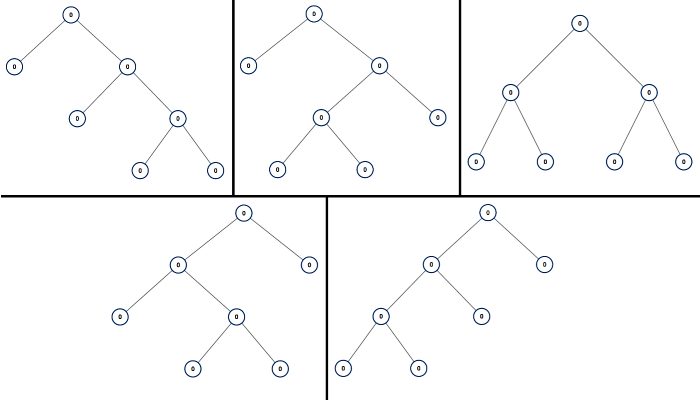# LeetCode Weekly Contest 99

https://leetcode.com/contest/weekly-contest-99

## Surface Area of 3D Shapes

On a N * N grid, we place some 1 * 1 * 1 cubes.

Each value v = grid[i][j] represents a tower of v cubes placed on top of grid cell (i, j).

Return the total surface area of the resulting shapes.

Example 1:

Example 2:

Example 3:

Example 4:

Example 5:

Note:

1. 1 <= N <= 50
2. 0 <= grid[i][j] <= 50

## Groups of Special-Equivalent Strings

You are given an array A of strings.

Two strings S and T are special-equivalent if after any number of moves, S == T.

A move consists of choosing two indices i and j with i % 2 == j % 2, and swapping S[i] with S[j].

Now, a group of special-equivalent strings from A is a non-empty subset of A such that any string not in A is not special-equivalent with any string in A.

Return the number of groups of special-equivalent strings from A.

Example 1:

Example 2:

Example 3:

Example 4:

Note:

1. 1 <= A.length <= 1000
2. 1 <= A[i].length <= 20
3. All A[i] have the same length.
4. All A[i] consist of only lowercase letters.

## All Possible Full Binary Trees

A full binary tree is a binary tree where each node has exactly 0 or 2 children.

Return a list of all possible full binary trees with N nodes. Each element of the answer is the root node of one possible tree.

Each node of each tree in the answer must have node.val = 0.

You may return the final list of trees in any order.

Example 1:Note:

1. 1 <= N <= 20

1. N为偶数时，不存在满二叉树
2. N==1时，独立的根结点可以形成一个满二叉树
3. N>=3时，根结点下有两棵子树，左子树的结点数可以为<N的任意奇数i，右子树的结点数则为N-1-i

## Maximum Frequency Stack

Implement FreqStack, a class which simulates the operation of a stack-like data structure.

FreqStack has two functions:

• push(int x), which pushes an integer x onto the stack.
• pop(), which removes and returns the most frequent element in the stack.
• If there is a tie for most frequent element, the element closest to the top of the stack is removed and returned.

Example 1:

Note:

1. Calls to FreqStack.push(int x) will be such that 0 <= x <= 10^9.
2. It is guaranteed that FreqStack.pop() won’t be called if the stack has zero elements.
3. The total number of FreqStack.push calls will not exceed 10000 in a single test case.
4. The total number of FreqStack.pop calls will not exceed 10000 in a single test case.
5. The total number of FreqStack.push and FreqStack.pop calls will not exceed 150000 across all test cases.